# J9九游会AG

TEL：0755-29126662

# Guide to testing the control loop in a Switched Mode Power Supply

Introduction

Frequency Response Analysis is widely recognised as the most effective way to test and quantify the transfer function of a power supply control loop and the SMPS demonstration system used by Newtons4th provides a convenient and effective way to illustrate this critical test.

While the fundamental principles of control loop testing and use of the PSM series analysers from Newtons4th are covered by our application documents, this guide aims to answer some common questions that are raised in relation to this application.

In order to answer these questions, we will use the power supply selected for our SMPS demonstration system to illustrate basic power supply techniques and some considerations that should be taken into account when testing almost any power supply design.

Questions

1. How is the control loop identified in a power supply if an original circuit diagram is not available?

2. How is the best location for the injection resistor selected?

3. What criteria are used to select the value of the injection resistor?

4. What is the principle of gain / phase margins and how can PSM results be verified?

Theory

As a general point of reference, it is useful to keep in mind the basic principal of a control loop and typical circuit layout reflecting the generic elements that will be found in most SMPS designs.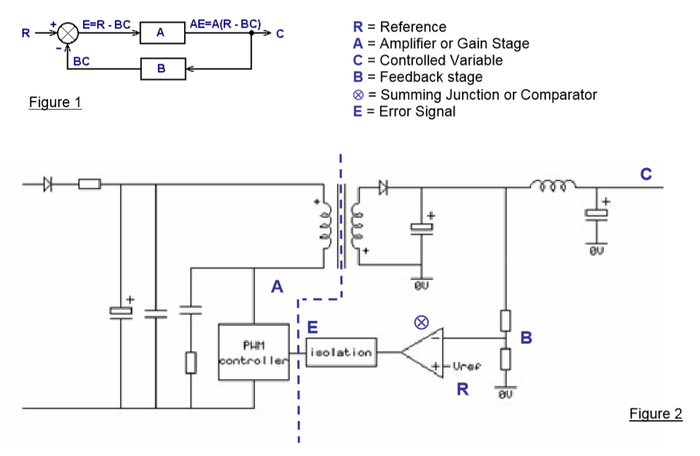1. Identifying the control loop

From figure 2, it can be seen that the key elements of a control loop are on the isolated side of the power supply and it is therefore this part of the circuit that we need to focus upon.

Given familiarity with general power supply principles, it is possible to look at a power supply and derive sufficient understanding to establish the basic architecture of the design. Here, we will look at the power supply used in the SMPS Demo system and using pictures of the board from the top and bottom, produce a circuit diagram of the output, feedback, comparator and isolation stages.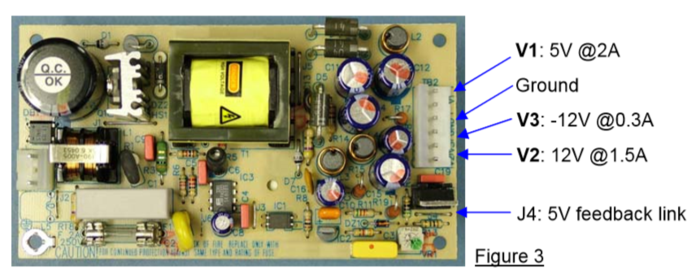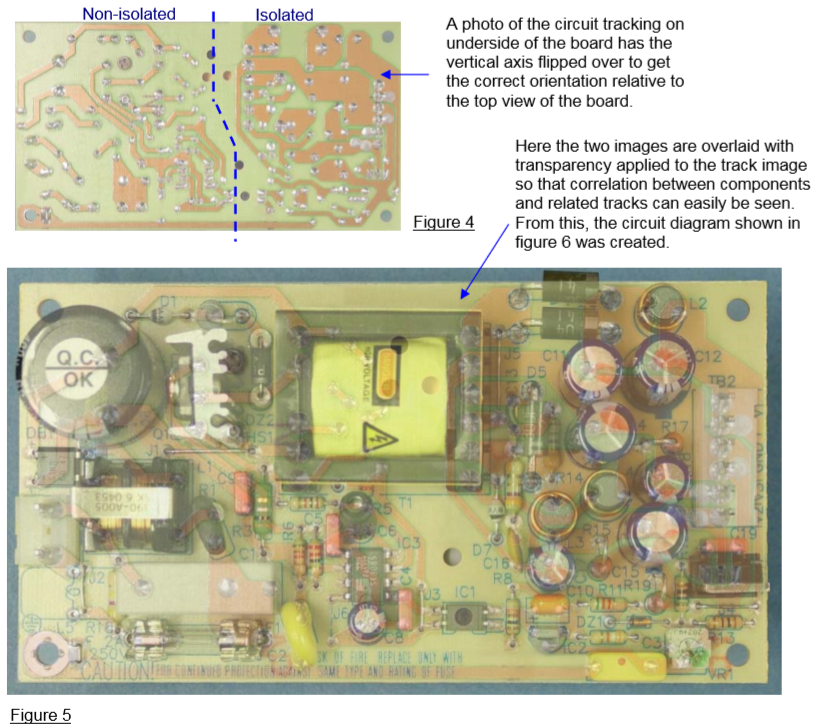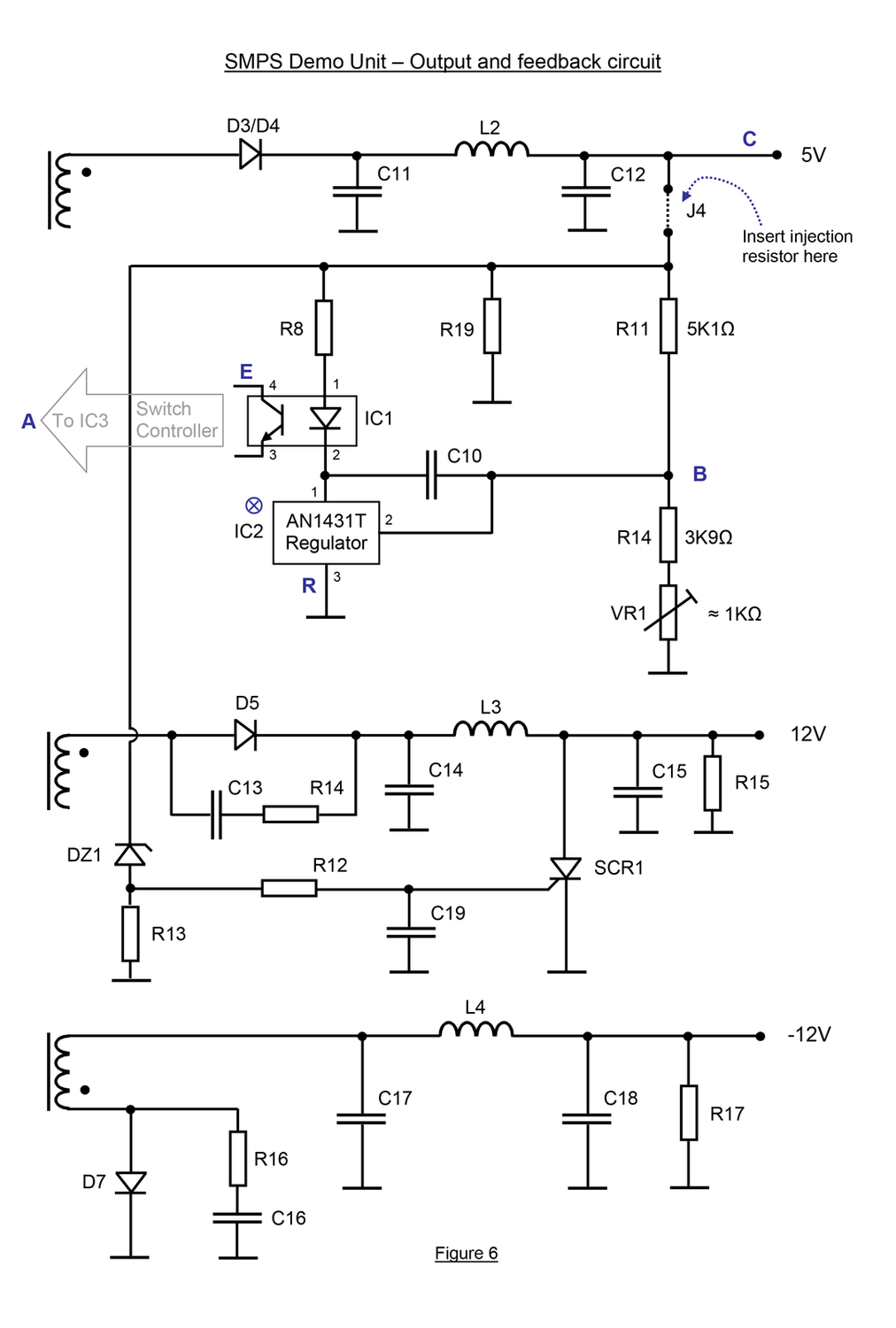Key components of the control loop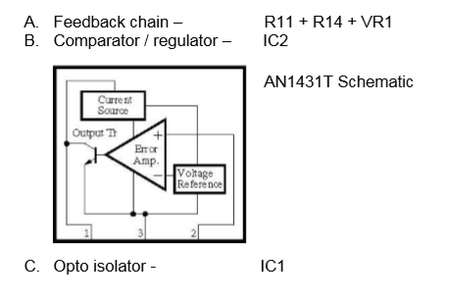2. Location of the injection resistor

The most effective analysis of a closed loop transfer function is achieved by placing a resistor (across which a disturbance signal can be generated and the relative gain / phase can be measured) into the loop at a point where the resistor itself will have minimal effect on the circuit.

It is best to select a high impedance part of the loop that is as close as possible to the main regulated output. In single output power supplies the point of connection is quite obvious but even with multi-output supplies like the SMPS demo unit, it does not take long to identify the fully regulated output and in most multi output SMPS units, it is the 5V output.

Looking directly at the power supply or at the diagram that has been produced from it, you should find the point in the circuit where there is a high impedance resistor chain to ground. This is usually easy to find because the feedback chain will be a low current path and therefore the resistance of the chain will always be relatively high.

In many power supplies, a jumper lead is included in the design that simplifies the connection of an injection resistor and this is true of the SMPS demo unit where ‘J4’ is ideal point to connect the injection resistor.

If no jumper lead is available, it is usually possible to lift one end of the resistor closest to the output in the feedback chain. For example, with a circuit similar to the one shown in figure 2, the injection resistor would be connected as follows: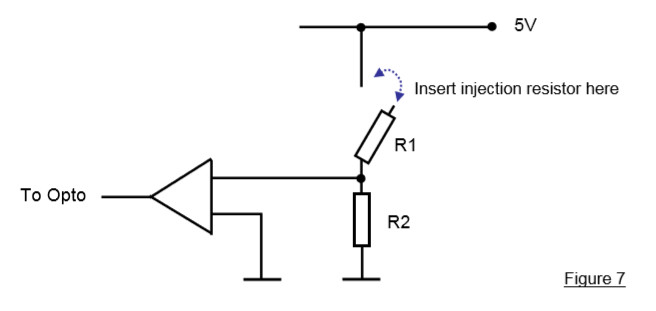3. Selection of the injection resistor value

The optimum value for the injection resistor will provide sufficient impedance to permit the isolated signal generator to achieve the desired disturbance signal while being small enough to have negligible effect on the circuit being tested.

The points that should be considered are:

A. Desired disturbance voltage - The ideal disturbance voltage will be sufficient for the measurement system to achieve stable results while being with the regulation voltage range of the power supply being tested. In the case of Newtons4th PSM analysers, stable measurements will usually be obtained with a signal level of 50mV or greater and as a general rule; the maximum signal level should be about 10% of the specified power supply output voltage (i.e. maximum 500mV for a 5V output).

B. Total resistance of the feedback chain - A typical SMPS may have a feedback chain of about 10K Ω and this is true of the SMPS demo unit which has a feedback chain comprising of 5K1 Ω (R11), 3K9 Ω (R14) and 1K Ω (VR1) giving 10 KΩ.

C. Resistor tolerance of the feedback chain or specified regulation of SMPS - Provided that the value of the injection resistor results in a change to the output voltage that is within the tolerance of the resistor chain or the basic accuracy of the power supply being tested, it can be considered to introduce no change to the power supply function.

In the SMPS demo system, a 10 Ω resistor is used. This is sufficient for the PSM generator to easily achieve a 100mV signal and is well within the working range of a 5V power supply. At 0.1% of the total feedback chain resistance, the injection resistor is much less than the feedback chain tolerance or the accuracy of the power supply.

4. Verification of Gain and Phase results

The objective of tests made in this application is to obtain a plot of loop gain over a selected frequency range and a plot of phase shift (sometimes referred to as ‘loop delay’) over the same frequency range. It is normal to present results in a Bode plot format and from these results; the overall stability of the control loop can be established by obtaining the Gain and Phase Margin. These margins are defined as follows:

Gain margin The gain difference from 0dB at the frequency where the phase difference is -360° (phase crossover frequency) Phase Margin The phase difference from -360° at the frequency where the gain is 0dB (gain crossover frequency)

The computation of theoretical gain and phase on complex circuits is possible using mathematical simulation but is not reliable when real world circuits incorporate parasitic components that are usually unknown at higher frequencies. Comparison of results on quite simple circuits that are modeled in a program like ‘Matlab‘ can be interesting but in practice, mathematical simulations are an unreliable reference for real power supplies with the uncertainly of component tolerance and the completely unknown elements of parasitic impedance associated with the circuit layout that has significant effect as the frequency of test is increased.

In practice however, it is possible to substantiate the gain and phase results presented by PSM series analysers because all results are derived from the basic elements of the voltage and phase difference at either side of the injection resistor. In addition to the computed values that are presented in the instrument display, the voltage measured on each point of a sweep and the phase angle between those voltages are available on the instrument display and are included in tabular results sent to a PC.

The following table illustrates typical results and how to verify them.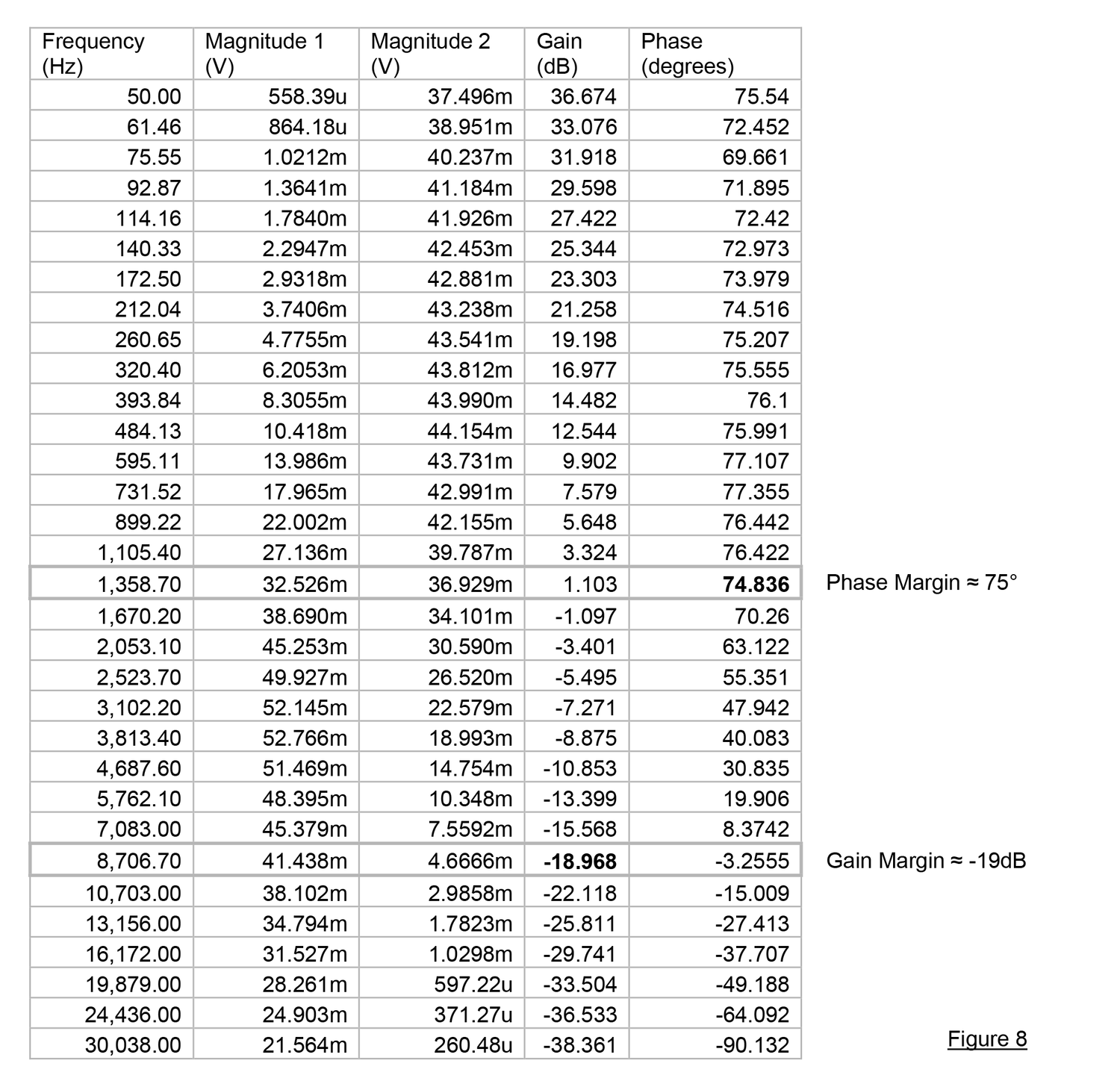Here it can be seen that the voltage magnitude measured on each channel is within the standard list of results presented by a PSM analyser. From these voltages, the expected value of Gain in DB can be derived from the equation:

dB = 20log (Vout / Vin)

So, using the measured values obtained at 50Hz:   20 log (37.496 x 10-3 / 558.39 x 10-6) = 36.5 dB

As in this case, very small differences may be seen between computed and presented dB values and this is because the computation within the PSM uses greater resolution than is presented in the table.

Comparison with external voltage and phase measurement instruments connected to the injection resistor would in theory provide an independent cross reference to the measured results but in practice, very few measurement instruments provide sufficient accuracy or dynamic range to achieve results of comparable precision, so each PSM instrument is supplied with a traceable certificate of accuracy.

With its ability to extract specific frequency components that are immersed in noise and measure gain and phase components with great precision, the PSM has become a recognised standard in this application.

Frequency

Shenzhen XingliGao Electronics Technology Co., Ltd.